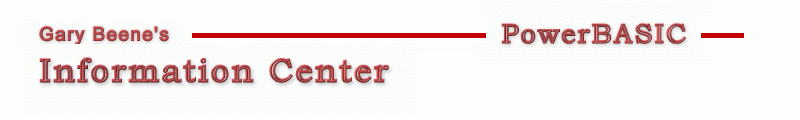## BMP Tutor - Create *.bmp by Code

Category: BMP Tutor Series (API)

Date: 03-28-2012

'It's possible to create a 24-bit bitmap by simply providing values for
'You must also provide a byte array that contains the pixel color values.
'With all 3 in hand (2 headers + 1 byte array), you can simply use
'PowerBASIC OPEN/PUT statements to save the information to a *.bmp file.

'The array will consist of color values in BGR format. Each row
'(also called scanlines) are padded with empty bytes to ensure
'that each scanline will contain a multiple of 4 bytes.

'Note that with 24bit bitmaps, no palette information is required.
'All of the color informationi is in the pixel byte array.

'Bitmap files (*.bmp) contain DIBs - device independent bitmaps.

'Primary Code:
'An excerpt of the code if provided here. See the compilable example
'below for more details.
'1. Create the pixel data
padding  = (4 - (w*3) Mod 4) Mod 4        'padding for each scanline
Dim Buffer(w*3+padding-1,h-1) As Byte     'pixel color array data (0-based array)
For y = 0 to h-1                  'each row
For x = 0 To w*3-1            'each pixel in each row (3 bytes per pixel, BGR byte order, ignores padding bytes)
'edit each BGR byte component however you want
Next x
Next y

bmfh.bfType        = CVI("BM")    '&H4d42 = CVI("BM")
...

info.biSize          = 40         '40 = SizeOf(BITMAPINFOHEADER)
...

'Compilable Example:
'This shows how to create bitmap data entirely by code. It fills in
'creates a Byte array to hold the image color data and then programatically
'modifies the content of the array.
#Compiler PBWin 9, PBWin 10
#Compile EXE
#Dim All
#Include "Win32API.inc"
#Resource "gbsnippets.pbr"

Global hDlg, hGraphic, hBMP, DC_graphic, DC_BMP as DWord

Function PBMain() As Long
Dialog New Pixels, 0, "Test Code",300,300,200,200, %WS_OverlappedWindow To hDlg
Control Add Button, hDlg, 100,"Create Bitmap", 20,10,150,20
Dialog Show Modal hDlg Call DlgProc
End Function

CallBack Function DlgProc() As Long
If CB.Msg = %WM_Command AND CB.Ctl = 100 AND CB.Ctlmsg = %BN_Clicked Then
Local w, h As Long
w = 100 : h = 100
CreateBitmapFromScratchAndSave w,h,"test.bmp"
'display the results in a Graphic Control
Control Add Graphic, hDlg, 900, "", 50,50,w,h
Graphic Attach hDlg, 900 : Graphic Render "test.bmp", (0,0)-(w-1,h-1) :
End If
End Function

Sub CreateBitmapFromScratchAndSave(w As Long, h as Long, bmpfile As String)

'Create buffer (byte array) to hold the BGR pixel color values
padding  = (4 - (w*3) Mod 4) Mod 4
Dim Buffer(w*3+padding-1,h-1) As Byte     '0-based array

'Manually edit some of the bytes
If 0 Then
For y = 0 to h-1                  'each row
For x = 0 To w*3-1            '1 byte at a time, 1 pixel = 3 bytes, BGR byte order, ignores padding bytes
If y <= (h-1)/2 Then Buffer(x,y) = 64 Else Buffer(x,y) = 128   'top half darker RGB = 64,64,64 vs 128,128,128
Next x
Next y

'Alternate way to manually edit some of the bytes
Else
For y = 0 to h-1                  'each row
For x = 0 To w*3-3 Step 3     '3 bytes at a time, 1 pixel = 3 bytes, BGR byte order, ignores padding bytes)
If y <= (h-1)/2 Then
'bottom half of image
Buffer(x,y)   = 0       'blue
Buffer(x+1,y) = 255     'green
Buffer(x+2,y) = 0       'red
Else
'top half of image
Buffer(x,y)   = 0       'blue
Buffer(x+1,y) = 0       'green
Buffer(x+2,y) = 255     'red
End If
Next x
Next y
End If

bmfh.bfType        = CVI("BM")    '&H4d42 = CVI("BM")
bmfh.bfReserved1   = 0
bmfh.bfReserved2   = 0

info.biWidth         = w
info.biHeight        = h
info.biPlanes        = 1         'must be 1
info.biBitCount      = 24        '24 or 32
info.biCompression   = %BI_RGB   'must be %BI_RGB (uncompressed)
info.biSizeImage     = 0         'zero for %BI_RGB images
info.biXPelsPerMeter = 0         'zero (device-specific value)
info.biYPelsPerMeter = 0         'zero (device-specific value)
info.biClrUsed       = 0         'no palette so set to zero
info.biClrImportant  = 0         'zero means all colors are important

'Open output file
Open bmpfile For Binary as #1